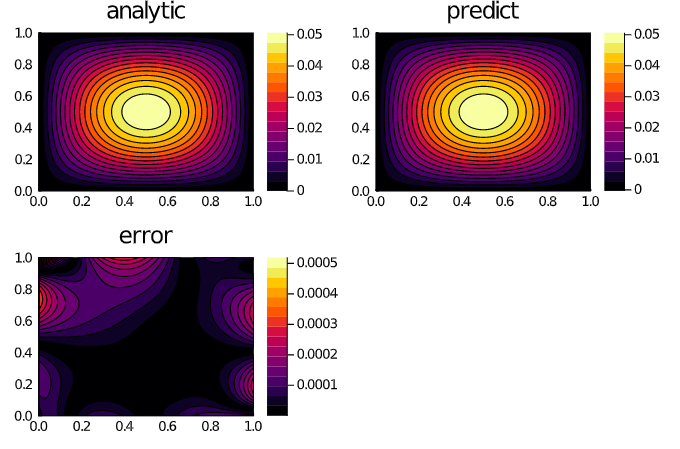# NeuralPDE

NeuralPDE.jl is a solver package which consists of neural network solvers for partial differential equations using physics-informed neural networks (PINNs). This package utilizes neural stochastic differential equations to solve PDEs at a greatly increased generality compared with classical methods.

## Installation

Assuming that you already have Julia correctly installed, it suffices to install NeuralPDE.jl in the standard way, that is, by typing ] add NeuralPDE. Note: to exit the Pkg REPL-mode, just press Backspace or Ctrl + C.

## Tutorials and Documentation

For information on using the package, see the stable documentation. Use the in-development documentation for the version of the documentation, which contains the unreleased features.

## Features

• Physics-Informed Neural Networks for ODE, SDE, RODE, and PDE solving
• Ability to define extra loss functions to mix xDE solving with data fitting (scientific machine learning)
• Automated construction of Physics-Informed loss functions from a high level symbolic interface
• Integrated logging suite for handling connections to TensorBoard
• Handling of (partial) integro-differential equations and various stochastic equations
• Specialized forms for solving ODEProblems with neural networks
• Compatability with Flux.jl and Lux.jl for all of the GPU-powered machine learning layers available from those libraries.
• Compatability with NeuralOperators.jl for mixing DeepONets and other neural operators (Fourier Neural Operators, Graph Neural Operators, etc.) with physics-informed loss functions

## Example: Solving 2D Poisson Equation via Physics-Informed Neural Networks

using NeuralPDE, Lux, ModelingToolkit, Optimization
import ModelingToolkit: Interval, infimum, supremum

@parameters x y
@variables u(..)
Dxx = Differential(x)^2
Dyy = Differential(y)^2

# 2D PDE
eq  = Dxx(u(x,y)) + Dyy(u(x,y)) ~ -sin(pi*x)*sin(pi*y)

# Boundary conditions
bcs = [u(0,y) ~ 0.0, u(1,y) ~ 0,
u(x,0) ~ 0.0, u(x,1) ~ 0]
# Space and time domains
domains = [x ∈ Interval(0.0,1.0),
y ∈ Interval(0.0,1.0)]
# Discretization
dx = 0.1

# Neural network
dim = 2 # number of dimensions
chain = Lux.Chain(Dense(dim,16,Lux.σ),Dense(16,16,Flux.σ),Dense(16,1))

@named pde_system = PDESystem(eq,bcs,domains,[x,y],[u(x, y)])
prob = discretize(pde_system,discretization)

callback = function (p,l)
println("Current loss is: \$l")
return false
end

res = Optimization.solve(prob, ADAM(0.1); callback = callback, maxiters=4000)
prob = remake(prob,u0=res.minimizer)
res = Optimization.solve(prob, ADAM(0.01); callback = callback, maxiters=2000)
phi = discretization.phi


And some analysis:

xs,ys = [infimum(d.domain):dx/10:supremum(d.domain) for d in domains]
analytic_sol_func(x,y) = (sin(pi*x)*sin(pi*y))/(2pi^2)

u_predict = reshape([first(phi([x,y],res.minimizer)) for x in xs for y in ys],(length(xs),length(ys)))
u_real = reshape([analytic_sol_func(x,y) for x in xs for y in ys], (length(xs),length(ys)))
diff_u = abs.(u_predict .- u_real)

using Plots
p1 = plot(xs, ys, u_real, linetype=:contourf,title = "analytic");
p2 = plot(xs, ys, u_predict, linetype=:contourf,title = "predict");
p3 = plot(xs, ys, diff_u,linetype=:contourf,title = "error");
plot(p1,p2,p3)### Citation

@article{zubov2021neuralpde,
title={NeuralPDE: Automating Physics-Informed Neural Networks (PINNs) with Error Approximations},
author={Zubov, Kirill and McCarthy, Zoe and Ma, Yingbo and Calisto, Francesco and Pagliarino, Valerio and Azeglio, Simone and Bottero, Luca and Luj{\'a}n, Emmanuel and Sulzer, Valentin and Bharambe, Ashutosh and others},
journal={arXiv preprint arXiv:2107.09443},
year={2021}
}


Get A Weekly Email With Trending Projects For These Topics
No Spam. Unsubscribe easily at any time.
Machine Learning (40,892
Deep Learning (39,311
Network (37,433
Neural (16,506
Neural Network (16,107
Julia (14,021
Physics (5,354
Differential Equations (324
Ode (181
Partial Differential Equations (160
Pde (142
Scientific Machine Learning (141
Sciml (114
Ordinary Differential Equations (80
Pinn (31
Neural Differential Equations (27
Scientific Ai (6
Scientific Ml (6